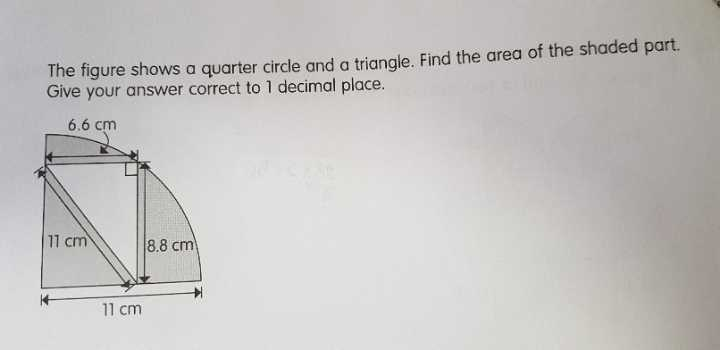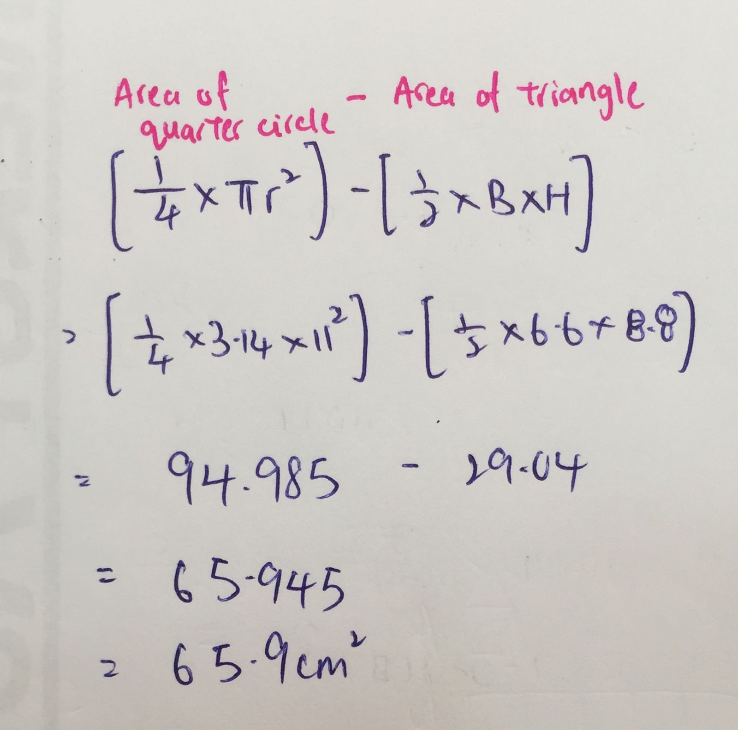# QuestionPls help me. Thanks!

area of the shaded part = [(1/4) x pi x 11 x 11] – [(1/2) x 8.8 x 6.6] = 65.9 (correct to 1 dp)

Ans : 65.9 sq cm (correct to 1 dp)

0 Replies 0 Likes0 Replies 0 Likes

Procedure:
1) Find area of the quarter circle.
2) Find area of triangle.
3) Shaded area = area of quarter circle – area of triangle.

Calculations:
1) Area of quarter circle: (3.14 x 11 x 11) ÷ 4 = 94.985cm²
(Note: area of quarter circle = area of circle ÷ 4)
2) Area of triangle: 1/2 x 6.6 x 8.8 = 29.04cm²
Therefore, shaded area is 94.985 – 29.04 = 65.945cm² = 65.9cm²

0 Replies 0 Likes